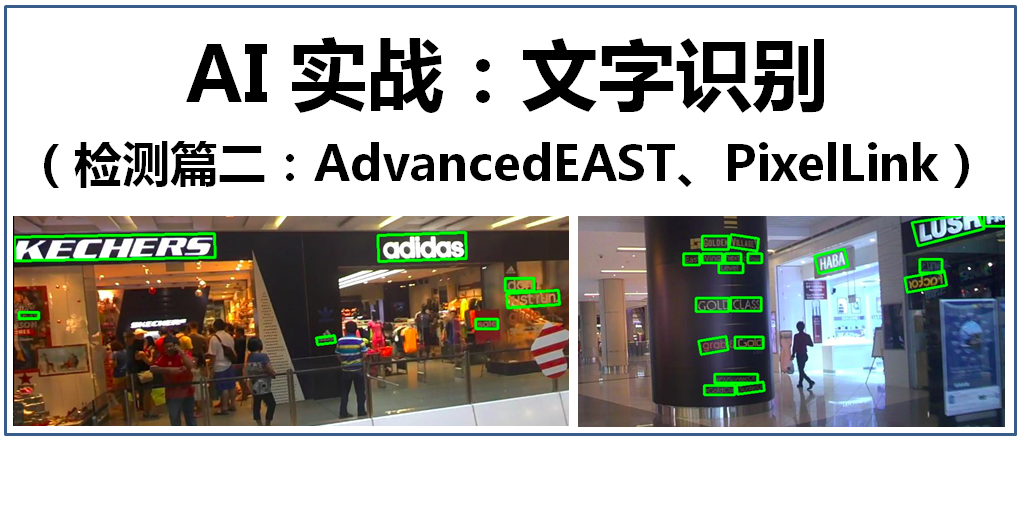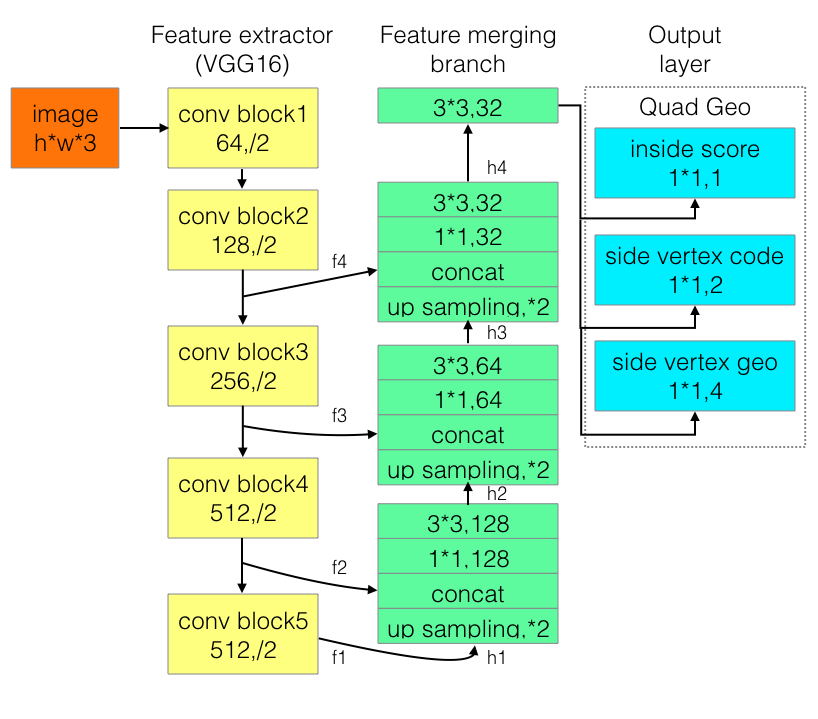（1）下载源代码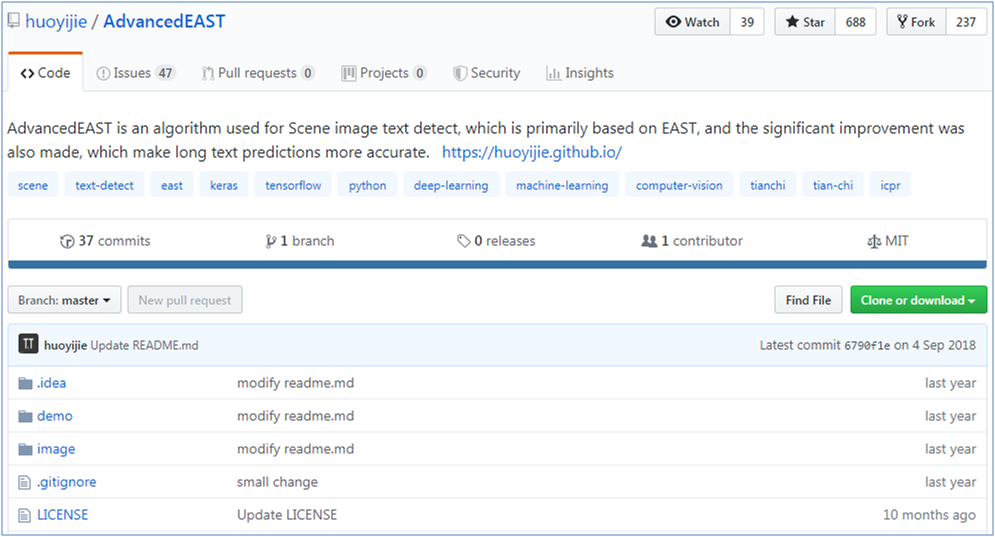``git clone https://github.com/huoyijie/AdvancedEAST.git``

（2）下载模型文件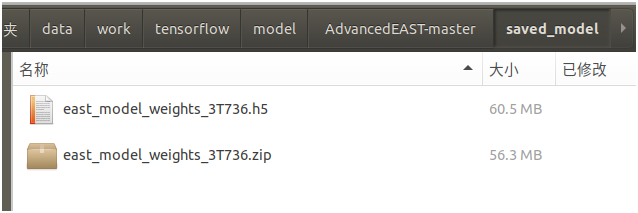``train_task_id = ‘3T736’``

``~/.keras/model``

（3）准备基础环境

• python 3.6.3+
• tensorflow-gpu 1.5.0+(or tensorflow 1.5.0+)
• keras 2.1.4+
• numpy 1.14.1+
• tqdm 4.19.7+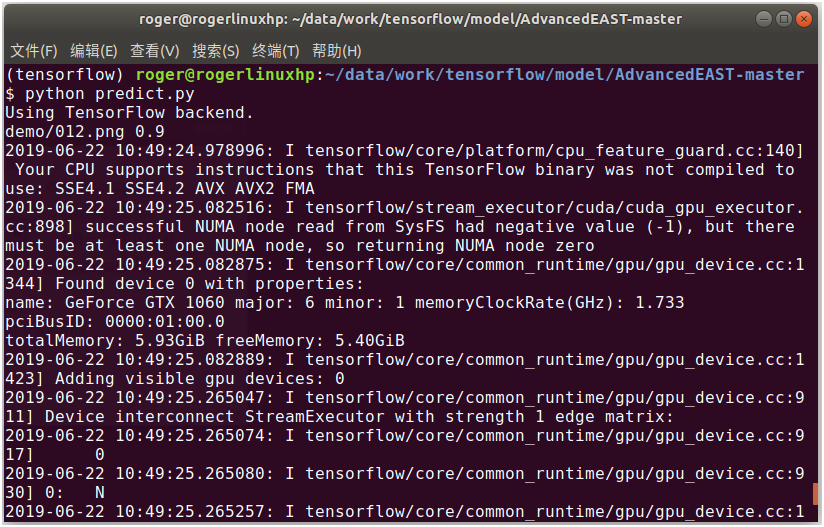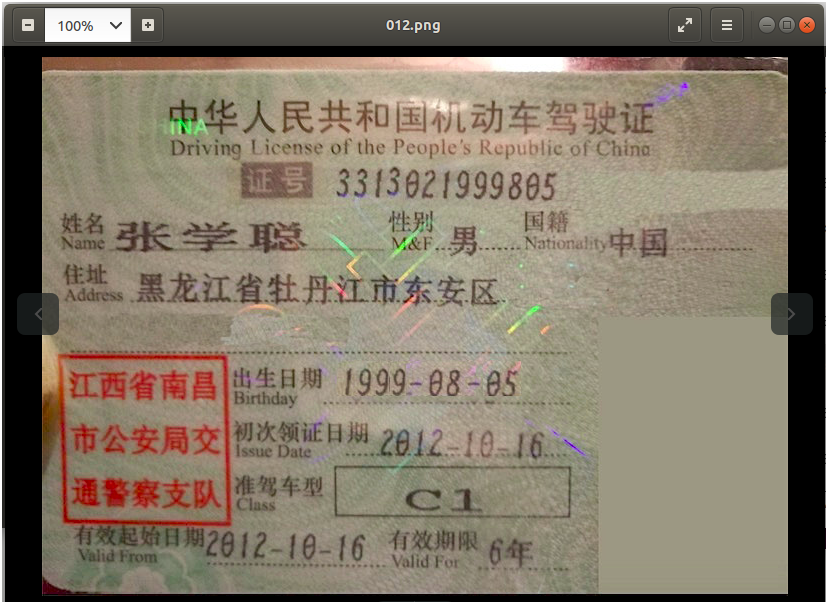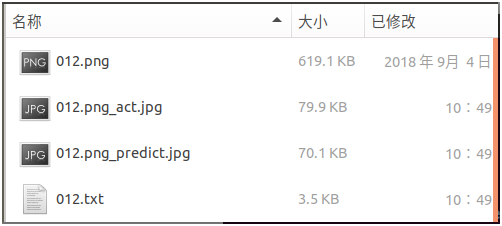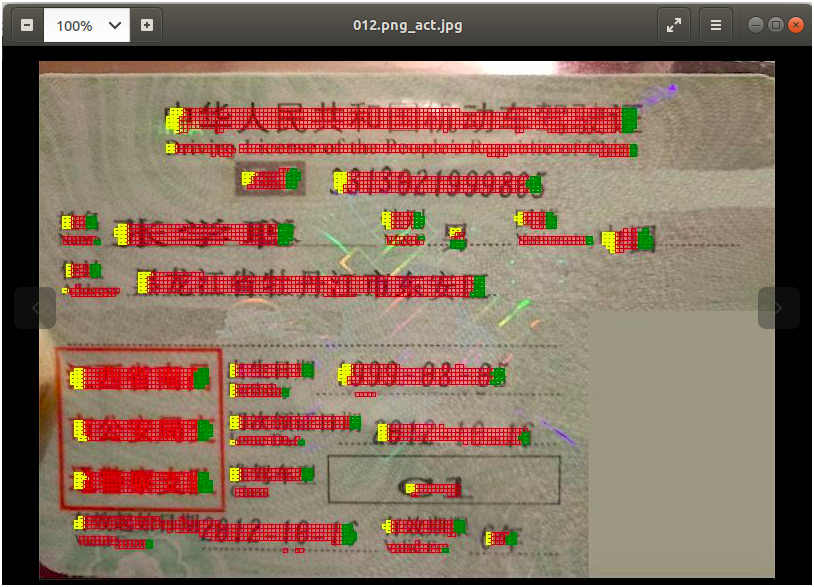012.png_predict.jpg是检测出最终文本框的圈定结果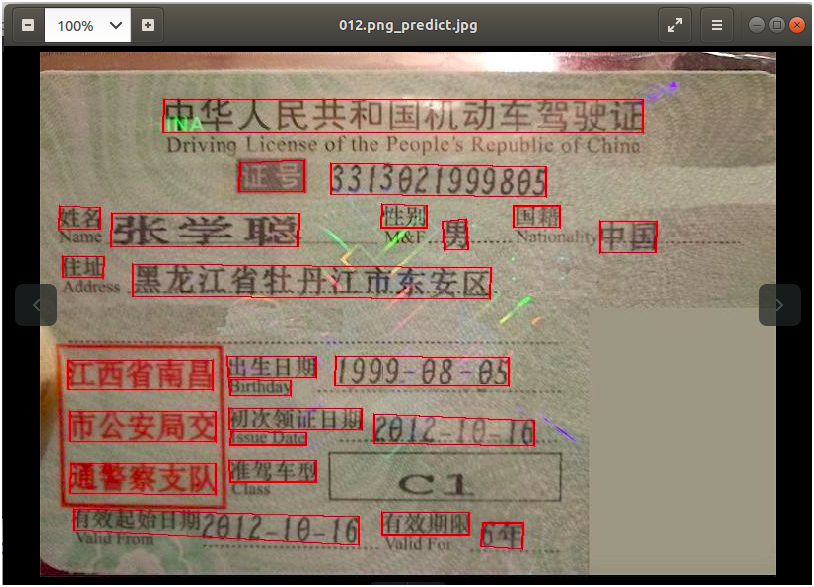012.txt是检测文本框的位置坐标（4个顶点）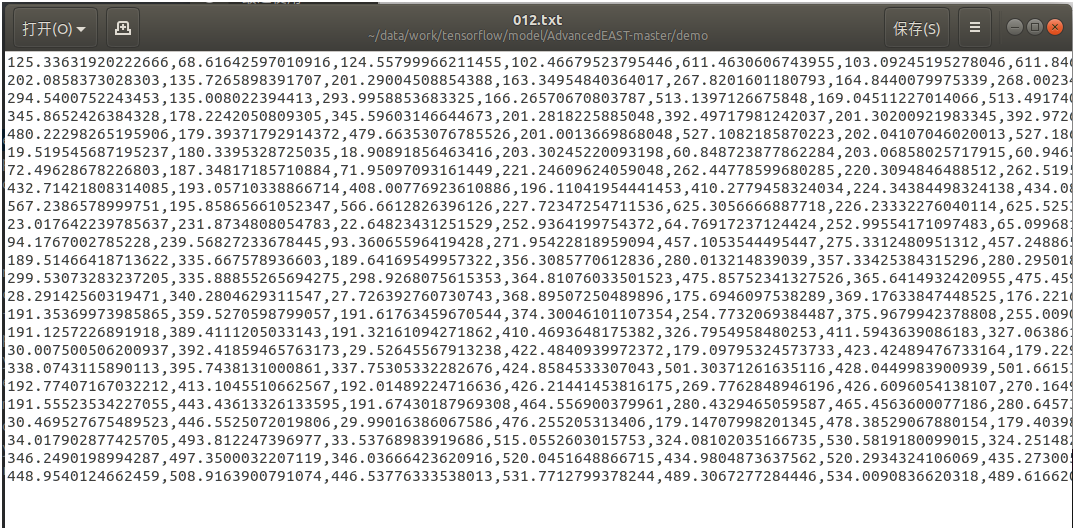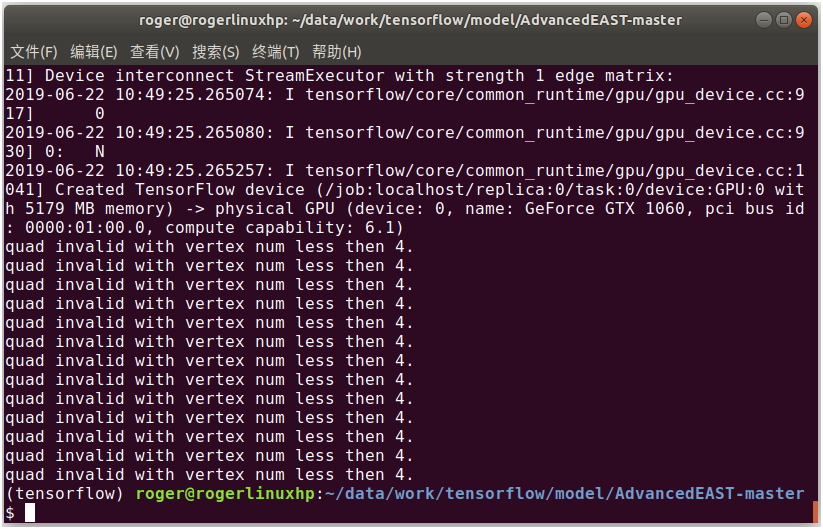``predict(east_detect, img_path, threshold, quiet=True)``

``python predict.py --path=/data/work/tensorflow/data/icdar_datasets/ICDAR2015/ch4_test_images/img_364.jpg --threshold=0.9``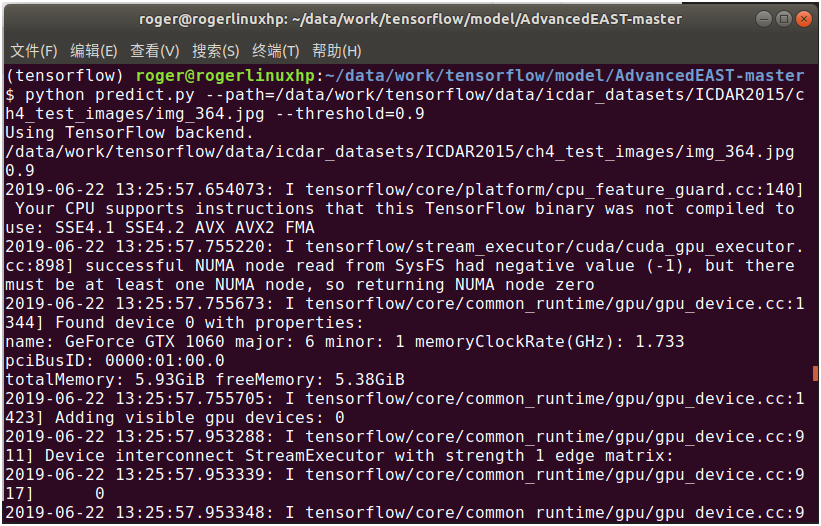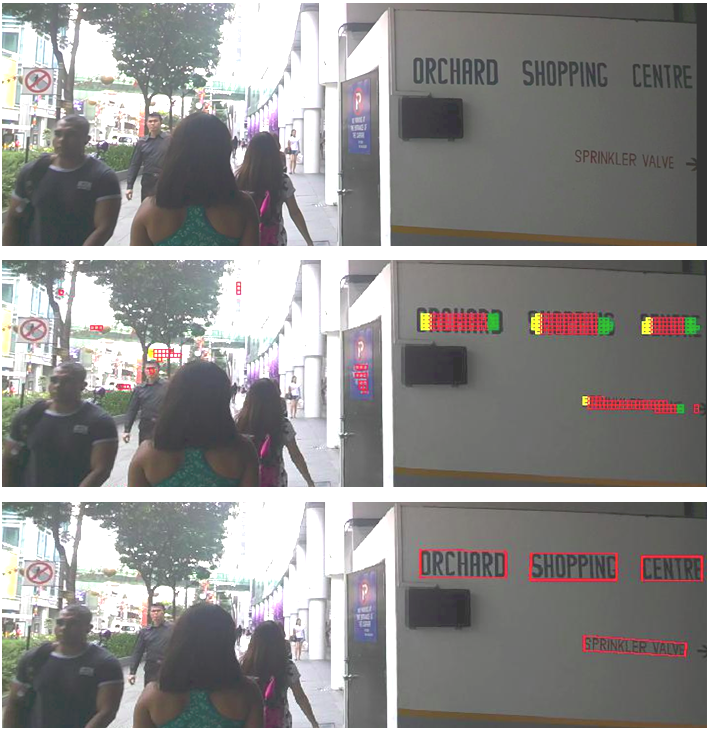``````# sigmoid 函数
def sigmoid(x):
return 1 / (1 + np.exp(-x))

def east_detect():
east = East()
east_detect = east.east_network()
return east_detect

# 返回：检测后文本框的位置信息
def text_detect(east_detect,img_path,pixel_threshold=0.9):
d_wight, d_height = resize_image(img, cfg.max_predict_img_size)
scale_ratio_w = d_wight / img.width
scale_ratio_h = d_height / img.height
img = img.resize((d_wight, d_height), Image.NEAREST).convert('RGB')
img = image.img_to_array(img)
img = preprocess_input(img, mode='tf')

x = np.expand_dims(img, axis=0)
y = east_detect.predict(x)

y = np.squeeze(y, axis=0)
y[:, :, :3] = sigmoid(y[:, :, :3])
cond = np.greater_equal(y[:, :, 0], pixel_threshold)
activation_pixels = np.where(cond)

bboxes = []
if np.amin(score) > 0:
rescaled_geo = geo / [scale_ratio_w, scale_ratio_h]
rescaled_geo_list = np.reshape(rescaled_geo, (8,)).tolist()
bboxes.append(rescaled_geo_list)

return bboxes``````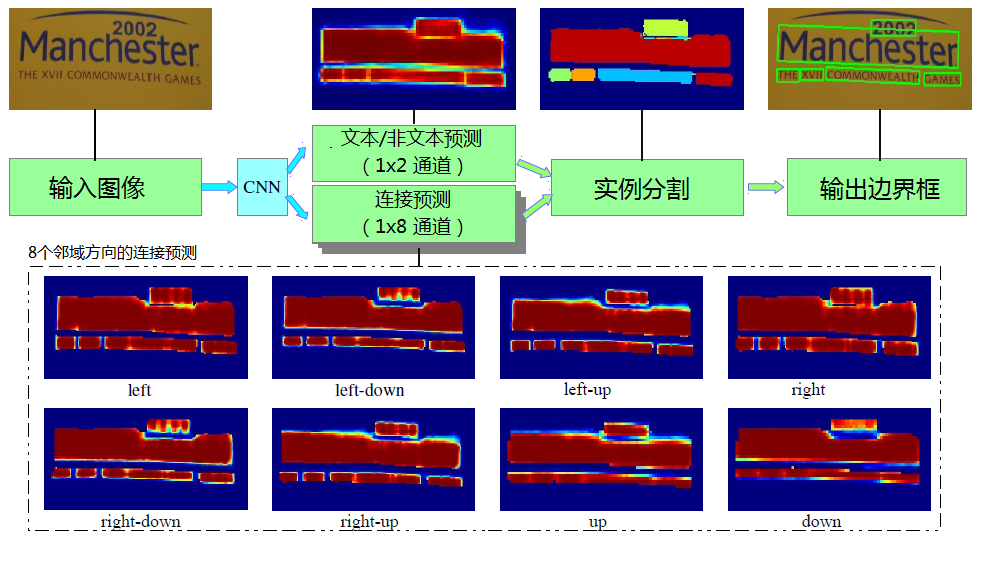（1）下载源代码和模型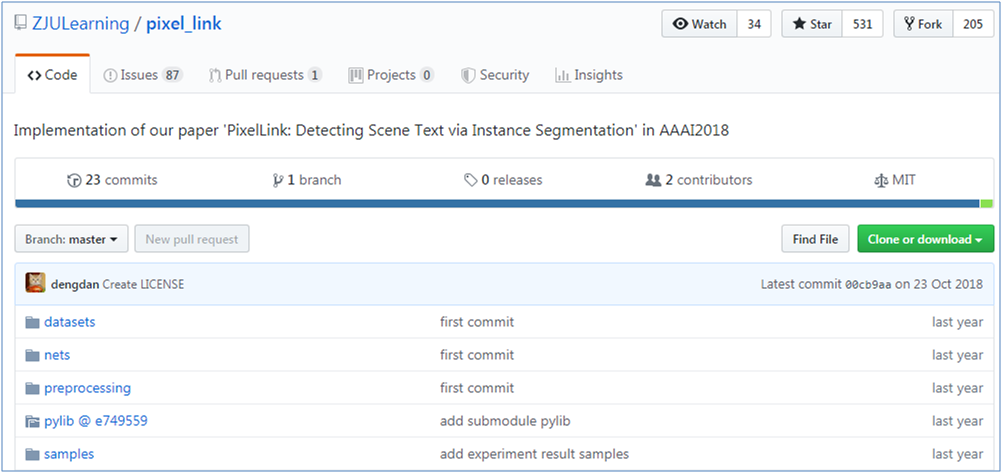``git clone https://github.com/ZJULearning/pixel_link.git``

``````import sys

``export PYTHONPATH=xx:\$PYTHONPATH``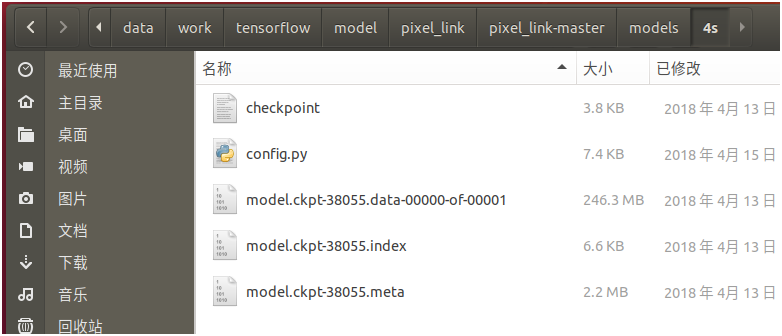（2）安装基础环境

``````name: pixel_link
channels:
- menpo
- https://mirrors.ustc.edu.cn/anaconda/pkgs/free
- https://mirrors.ustc.edu.cn/anaconda/pkgs/main
- defaults
dependencies:
- certifi=2016.2.28=py27_0
- cudatoolkit=7.5=2
- cudnn=5.1=0
- funcsigs=1.0.2=py27_0
- libprotobuf=3.4.0=0
- mkl=2017.0.3=0
- mock=2.0.0=py27_0
- numpy=1.12.1=py27_0
- openssl=1.0.2l=0
- pbr=1.10.0=py27_0
- pip=9.0.1=py27_1
- protobuf=3.4.0=py27_0
- python=2.7.13=0
- setuptools=36.4.0=py27_1
- six=1.10.0=py27_0
- sqlite=3.13.0=0
- tensorflow-gpu=1.1.0=np112py27_0
- tk=8.5.18=0
- werkzeug=0.12.2=py27_0
- wheel=0.29.0=py27_0
- zlib=1.2.11=0
- opencv=2.4.11=nppy27_0
- pip:
- backports.functools-lru-cache==1.5
- bottle==0.12.13
- cycler==0.10.0
- cython==0.28.2
- enum34==1.1.6
- kiwisolver==1.0.1
- matplotlib==2.2.2
- olefile==0.44
- pillow==4.3.0
- polygon2==2.0.8
- pyparsing==2.2.0
- python-dateutil==2.7.2
- pytz==2018.4
- setproctitle==1.1.10
- subprocess32==3.2.7
- tensorflow==1.1.0
- virtualenv==15.1.0``````

``conda env create --file pixel_link_env.txt``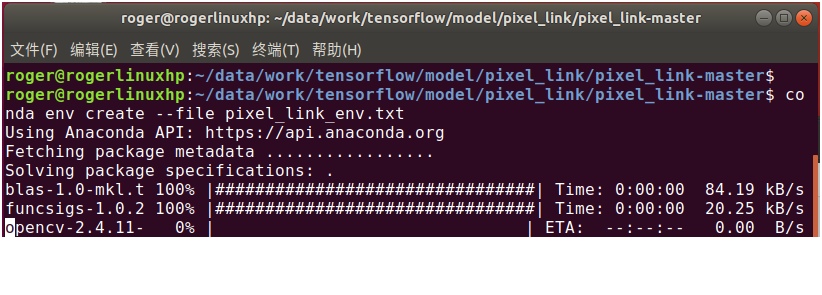``source activate pixel_link``

• 修改datasets/dataset_util.py第112行，在print后面加上括号
• 修改pylib/util/plt.py第174行，在print后面加上括号
• 修改pylib/util/img.py第8行，在print后面加上括号
• 修改pylib/util/proc.py第29行、第30行，在print后面加上括号。第35行，在raise后面加上括号
• 修改pylib/util/caffe_.py第29行、第46行、第47行、第50行，在print后面加上括号
• 修改pylib/util/tf.py第46行，将xrange改为range
• 修改models/4s/config.py第153行，将xrange改为range
• 由于在python3中没有cPickle，在pylib/util/io_.py中，第11行，将import cPickle as pkl改为import _pickle as pkl
• 由于在python3中commands已由subprocess代替，在pylib/util/io_.py中，第12行，将import commands改为import subprocess as commands。在pylib/util/cmd.py中，第4行，将import commands改为import subprocess as commands

``./scripts/test.sh \${GPU_ID} \${model_path}/model.ckpt-xxx \${image_dir}``

``./scripts/test.sh 0 models/4s/model.ckpt-38055 /data/work/tensorflow/data/icdar_datasets/ICDAR2015/ ch4_test_images``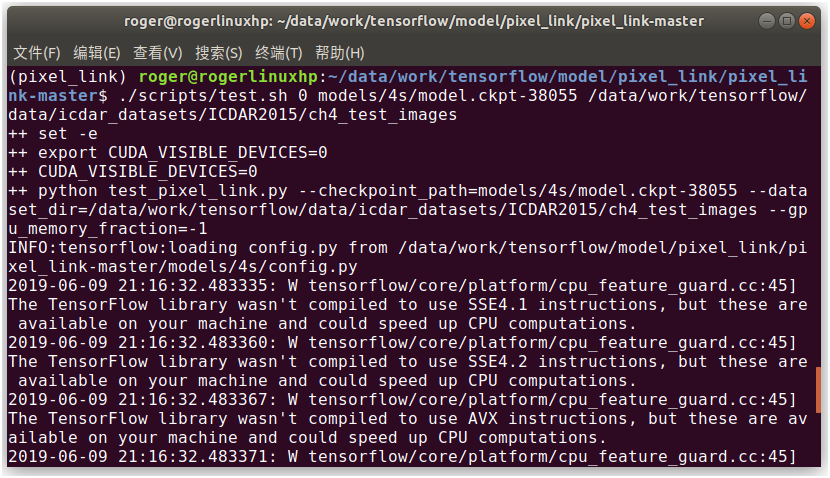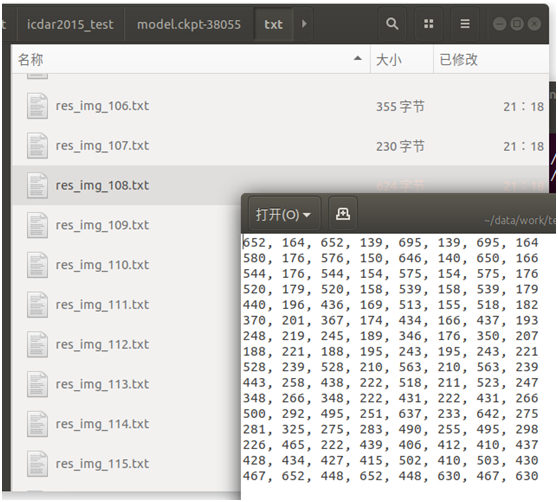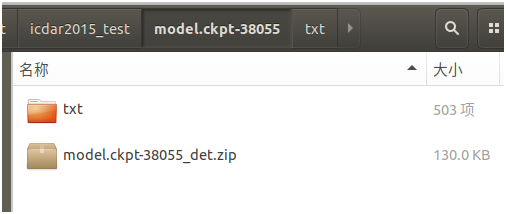``./scripts/vis.sh \${image_dir} \${det_dir}``

``./scripts/vis.sh /data/work/tensorflow/data/icdar_datasets/ICDAR2015/ch4_test_images models/4s/test/icdar2015_test/model.ckpt-38055/txt``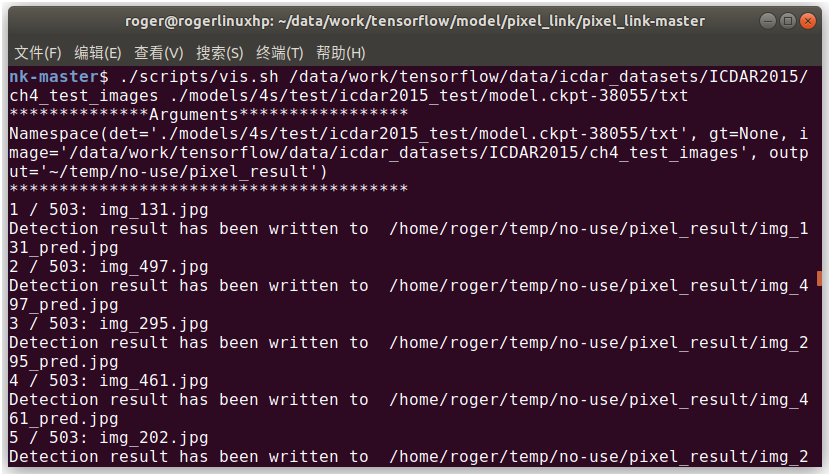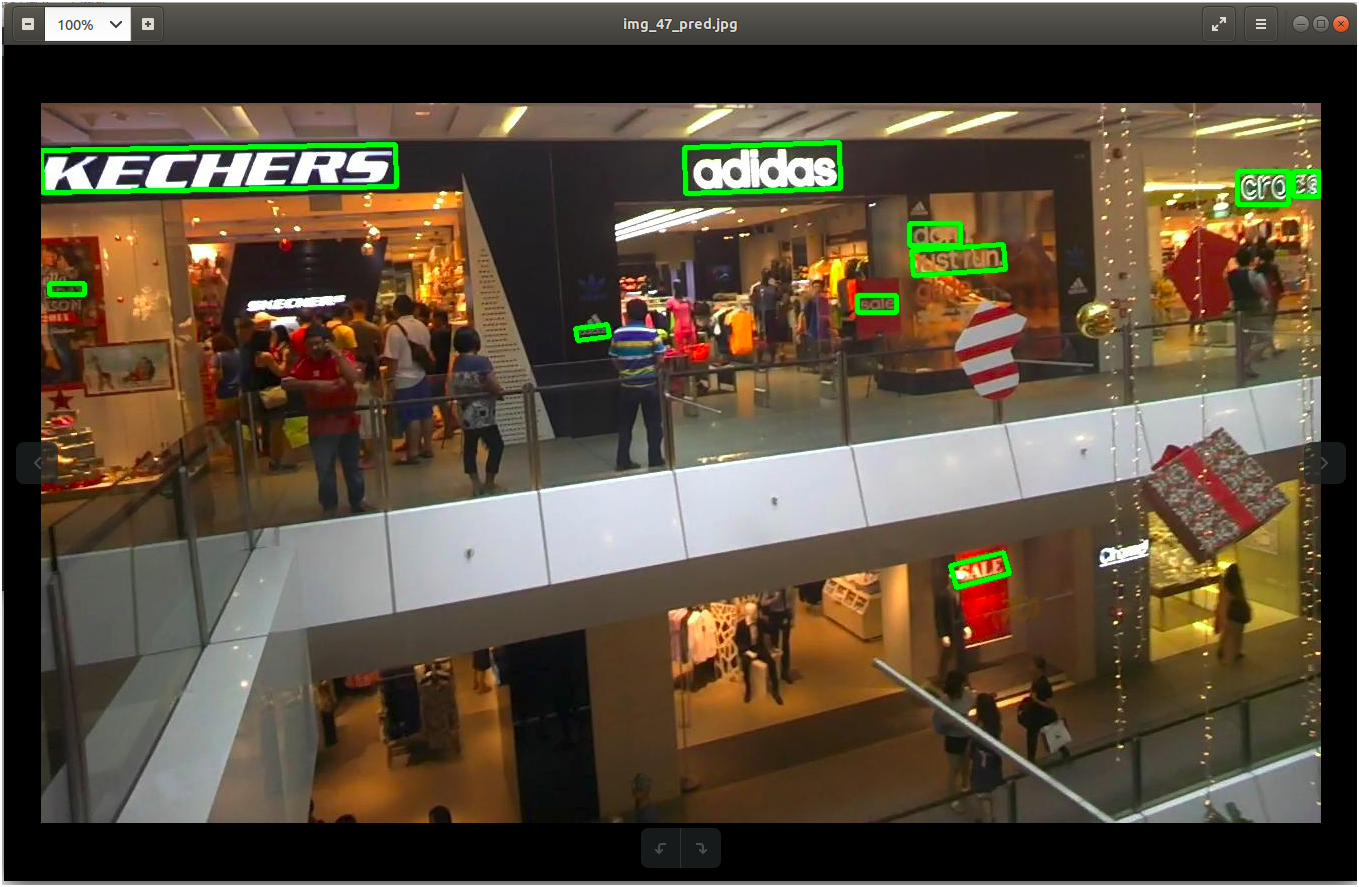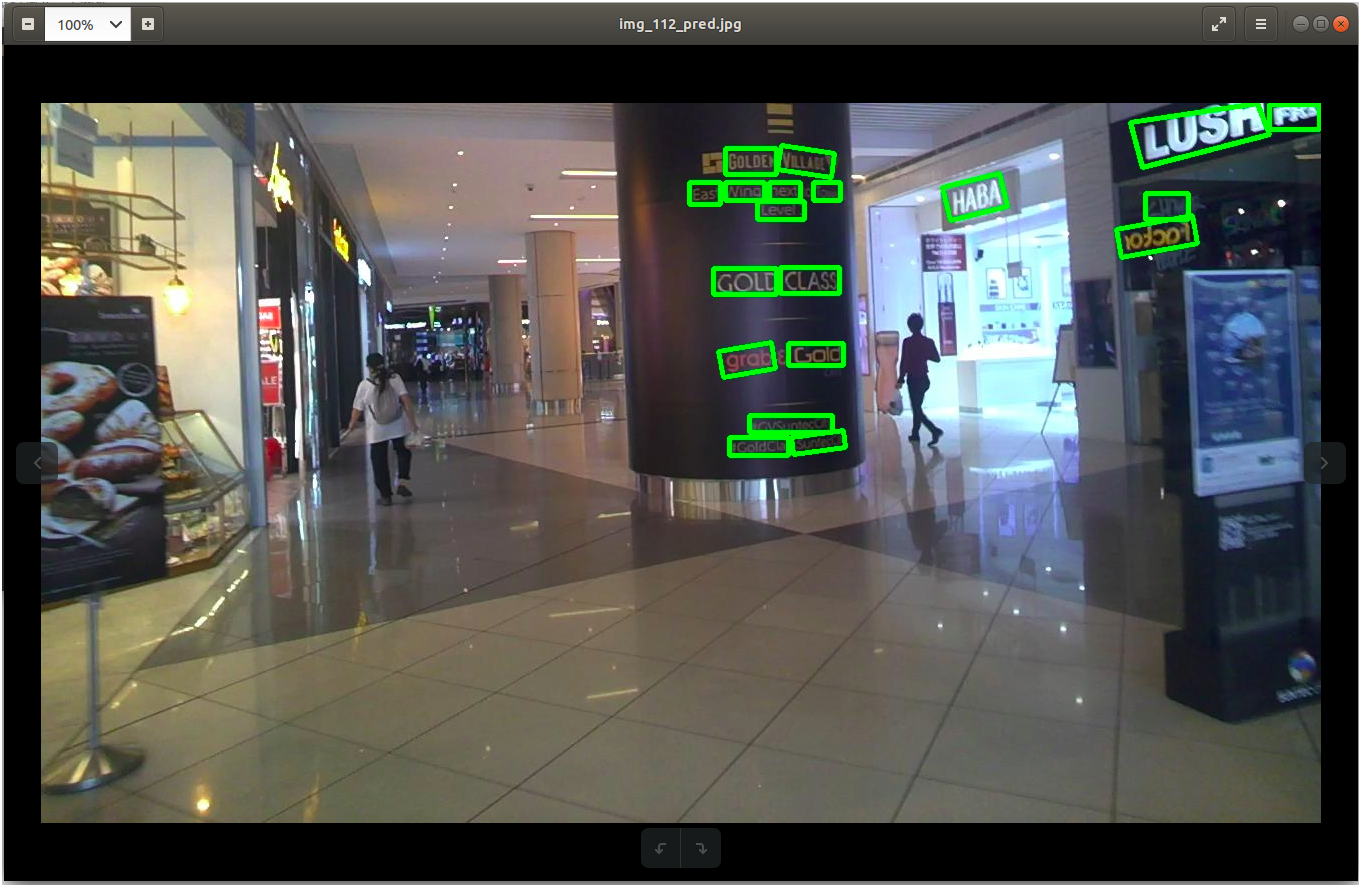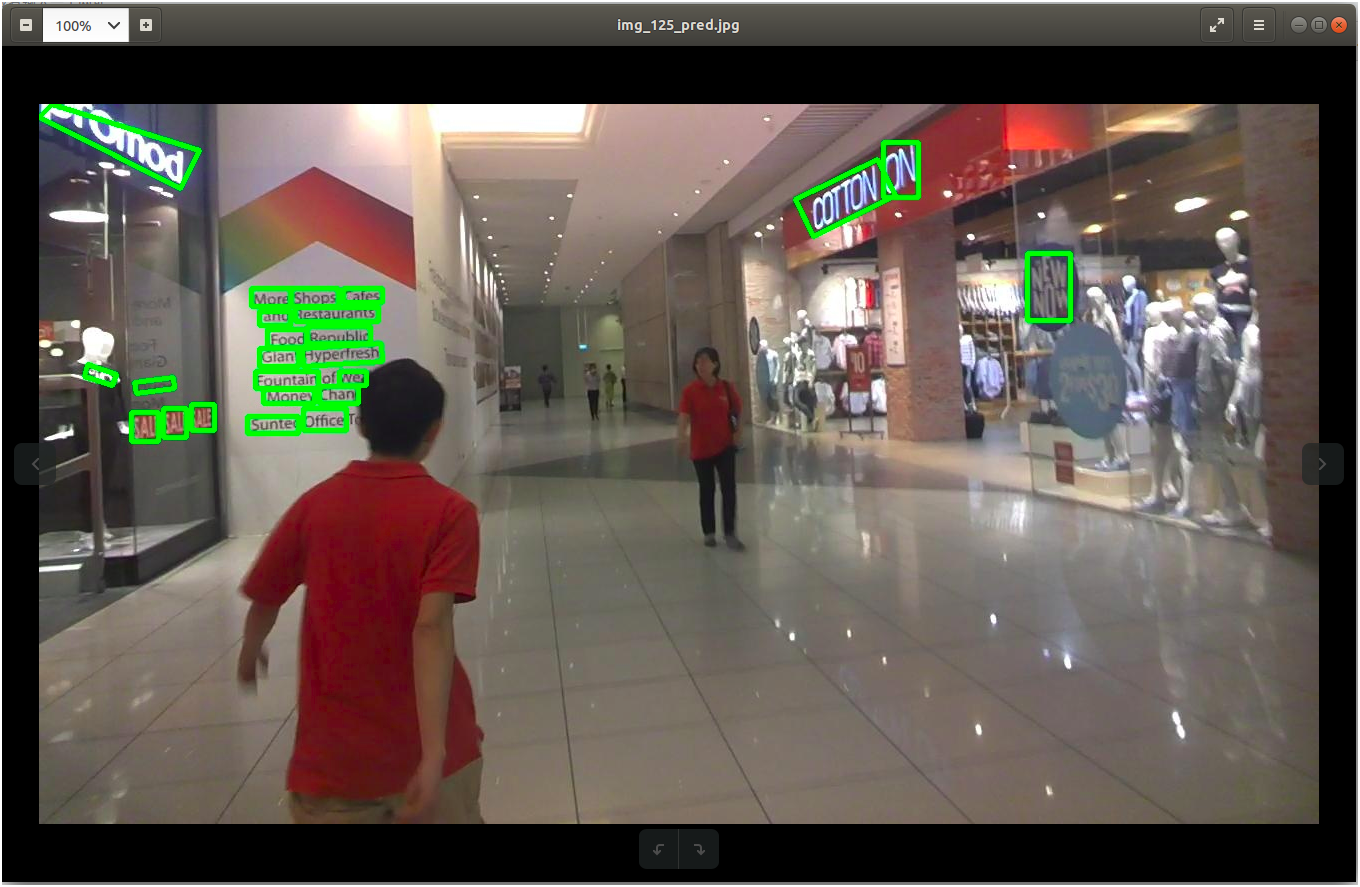``./scripts/test_any.sh \${GPU_ID} \${model_path}/model.ckpt-xxx \${image_dir}``

``./scripts/test_any.sh 0 models/4s/model.ckpt-38055 /data/work/tensorflow/data/icdar_datasets/ICDAR2015/ ch4_test_images``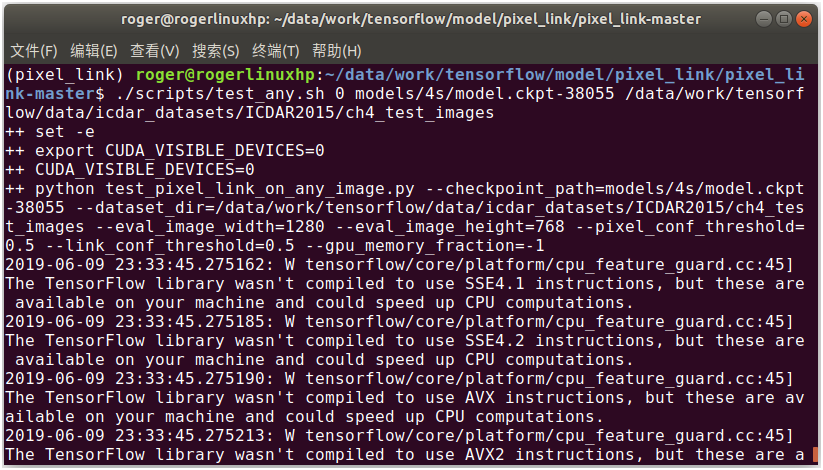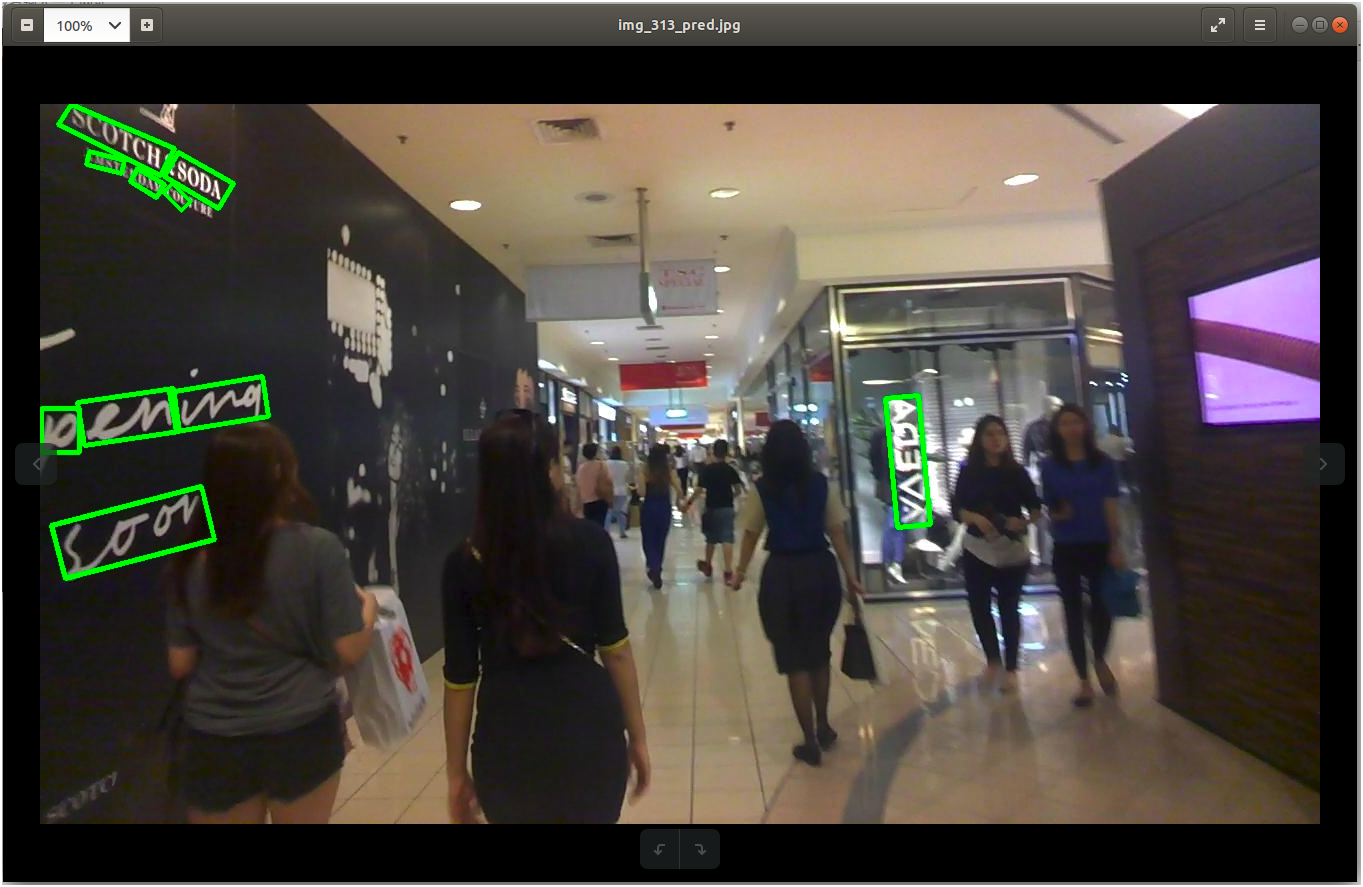``````# 模型参数
image_width = 1280
image_height = 768

# 配置初始化
def config_initialization():
image_shape = (image_height, image_width)

config.init_config(image_shape,
batch_size = 1,
pixel_conf_threshold = 0.8,
num_gpus = 1,
)

# 文本检测
def text_detect(img):
with tf.name_scope('eval'):
image = tf.placeholder(dtype=tf.int32, shape = [None, None, 3])
image_shape = tf.placeholder(dtype = tf.int32, shape = [3, ])
processed_image, _, _, _, _ = ssd_vgg_preprocessing.preprocess_image(image, None, None, None, None,
out_shape = config.image_shape,
data_format = config.data_format,
is_training = False)
b_image = tf.expand_dims(processed_image, axis = 0)
global_step = slim.get_or_create_global_step()

sess_config = tf.ConfigProto(log_device_placement = False, allow_soft_placement = True)
sess_config.gpu_options.allow_growth = True

saver = tf.train.Saver()

checkpoint = util.tf.get_latest_ckpt(checkpoint_dir)
bboxes = []

with tf.Session(config = sess_config) as sess:
saver.restore(sess, checkpoint)
image_data = img

feed_dict = {
image:image_data
})

return bboxes``````

a. 下载源代码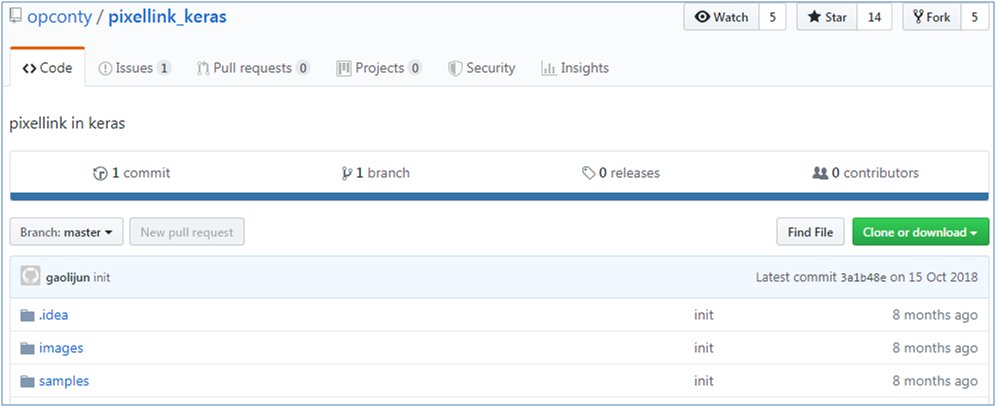``git clone https://github.com/opconty/pixellink_keras.git``

b. 下载预训练模型

c. 安装基础环境

``pip install imutils``

d. 运行模型

``python pixellink_eval.py``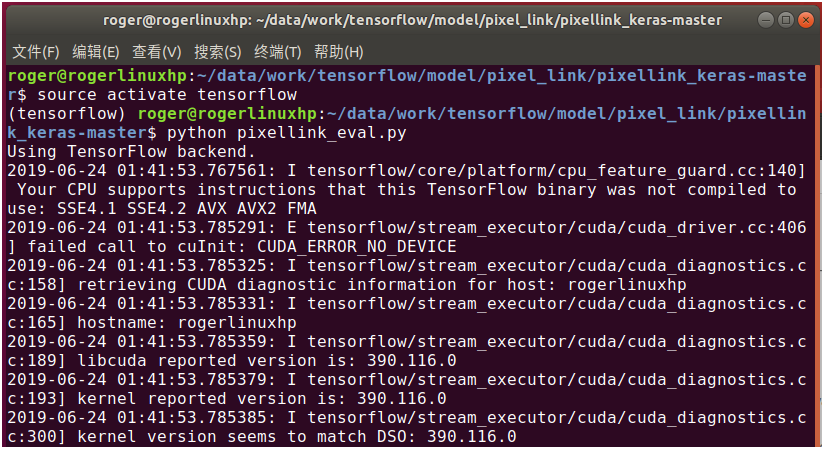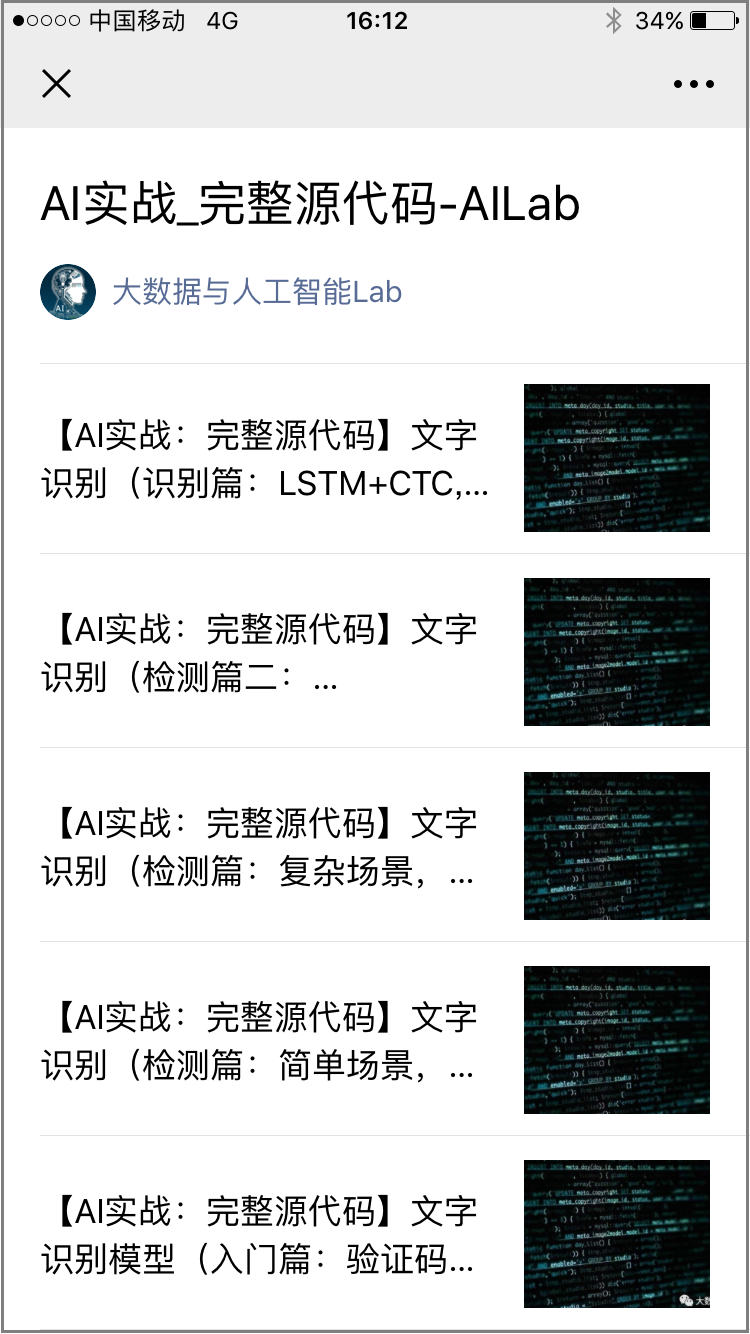1、AI 实战系列

2、大话深度学习系列

3、图解 AI 系列

4、AI 杂谈

5、大数据超详细系列### 雪饼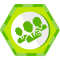### 评论(8)

【AI实战】手把手教你文字识别（识别篇：LSTM+CTC, CRNN, chineseocr方法）

07/07
0
0
【图解AI：动图】各种类型的卷积，你认全了吗？

06/20
0
0

05/27
0
6
【AI实战】手把手教你训练自己的目标检测模型（SSD篇）

2018/08/14
0
25
【AI实战】训练第一个AI模型：MNIST手写数字识别模型

2018/08/11
0
0

php 遇到 No input file specified的解决方法

（一）IIS Noinput file specified 方法一：改PHP.ini中的doc_root行，打开ini文件注释掉此行，然后重启IIS 方法二： 请修改php.ini 找到 ; cgi.force_redirect = 1 去掉前面分号，把后面的1...

chenhongjiang

5
0
MySQL 基础

6
0

1. 编写用于查询数据的功能函数 应用目录 下的 views.py 文件通常用于保存响应各种请求的函数或类 from django.shortcuts import renderfrom .models import BlogArticles# Create your ...

ZeroBit

5
0

21
0
jupyter部署安装

python373 -m ipykernel install --name python373 ipython kernelspec list sc create myjupyterservice binpath="D:\apply\Python373\Scripts\jupyter-notebook --config=V:/my_work/jupyt......

mbzhong

4
0